# Parity (mathematics)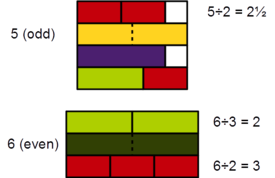Cuisenaire rods: 5 (yellow) cannot be evenly divided in 2 (red) by any 2 rods of the same color/length, while 6 (dark green) can be evenly divided in 2 by 3 (light green).

Parity is a mathematical term that describes the property of an integer's inclusion in one of two categories: even or odd. An integer is even if it is 'evenly divisible' by two (the old-fashioned term "evenly divisible" is now almost always shortened to "divisible") and odd if it is not even. For example, 6 is even because there is no remainder when dividing it by 2. By contrast, 3, 5, 7, 21 leave a remainder of 1 when divided by 2. Examples of even numbers include −4, 0, 8, and 1738. In particular, zero is an even number. Some examples of odd numbers are −5, 3, 9, and 73. Parity does not apply to non-integer numbers.

A formal definition of an even number is that it is an integer of the form n = 2k, where k is an integer; it can then be shown that an odd number is an integer of the form n = 2k + 1. This classification applies only to integers, i.e., non-integers like 1/2, 4.201, or infinity are neither even nor odd.

The sets of even and odd numbers can be defined as following:

• Even• OddA number (i.e., integer) expressed in the decimal numeral system is even or odd according to whether its last digit is even or odd. That is, if the last digit is 1, 3, 5, 7, or 9, then it is odd; otherwise it is even. The same idea will work using any even base. In particular, a number expressed in the binary numeral system is odd if its last digit is 1 and even if its last digit is 0. In an odd base, the number is even according to the sum of its digits it is even if and only if the sum of its digits is even.

## Arithmetic on even and odd numbers

The following laws can be verified using the properties of divisibility. They are a special case of rules in modular arithmetic, and are commonly used to check if an equality is likely to be correct by testing the parity of each side. As with ordinary arithmetic, multiplication and addition are commutative and associative in modulo 2 arithmetic, and multiplication is distributive over addition. However, subtraction in modulo 2 is identical to addition, so subtraction also possesses these properties, which is not true for normal integer arithmetic.

• even ± even = even;
• even ± odd = odd;
• odd ± odd = even;

Rules analogous to these for divisibility by 9 are used in the method of casting out nines.

### Multiplication

• even × even = even;
• even × odd = even;
• odd × odd = odd.

The structure ({even, odd}, +, ×) is in fact a field with just two elements.

### Division

The division of two whole numbers does not necessarily result in a whole number. For example, 1 divided by 4 equals 1/4, which is neither even nor odd, since the concepts even and odd apply only to integers. But when the quotient is an integer, it will be even if and only if the dividend has more factors of two than the divisor.

## History

The ancient Greeks considered 1, the monad, to be neither fully odd nor fully even. Some of this sentiment survived into the 19th century: Friedrich Wilhelm August Fröbel's 1826 The Education of Man instructs the teacher to drill students with the claim that 1 is neither even nor odd, to which Fröbel attaches the philosophical afterthought,

It is well to direct the pupil's attention here at once to a great far-reaching law of nature and of thought. It is this, that between two relatively different things or ideas there stands always a third, in a sort of balance, seeming to unite the two. Thus, there is here between odd and even numbers one number (one) which is neither of the two. Similarly, in form, the right angle stands between the acute and obtuse angles; and in language, the semi-vowels or aspirants between the mutes and vowels. A thoughtful teacher and a pupil taught to think for himself can scarcely help noticing this and other important laws.

## Higher mathematics

### Higher dimensions and more general classes of numbers

 a b c d e f g h 8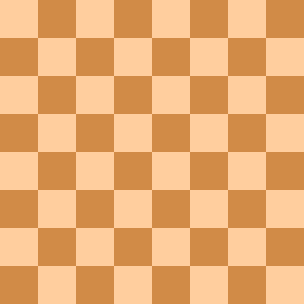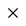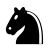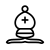8 7 7 6 6 5 5 4 4 3 3 2 2 1 1 a b c d e f g h
The two white bishops are confined to squares of opposite parity; the black knight can only jump to squares of alternating parity.

Integer coordinates of points in Euclidean spaces of two or more dimensions also have a parity, usually defined as the parity of the sum of the coordinates. For instance, the face-centered cubic lattice and its higher-dimensional generalizations, the Dn lattices, consist of all of the integer points whose sum of coordinates is even. This feature manifests itself in chess, where the parity of a square is indicated by its color: bishops are constrained to squares of the same parity; knights alternate parity between moves. This form of parity was famously used to solve the mutilated chessboard problem: if two opposite corner squares are removed from a chessboard, then the remaining board cannot be covered by dominoes, because each domino covers one square of each parity and there are two more squares of one parity than of the other.

The parity of an ordinal number may be defined to be even if the number is a limit ordinal, or a limit ordinal plus a finite even number, and odd otherwise.

Let R be a commutative ring and let I be an ideal of R whose index is 2. Elements of the cosetmaybe called even, while elements of the cosetmaybe called odd. As an example, let R=Z(2) be the localization of Z at the prime ideal (2). Then an element of R is even or odd if and only if its numerator is so in Z.

### Number theory

The even numbers form an ideal in the ring of integers, but the odd numbers do not this is clear from the fact that the identity element for addition, zero, is an element of the even numbers only. An integer is even if it is congruent to 0 modulo this ideal, in other words if it is congruent to 0 modulo 2, and odd if it is congruent to 1 modulo 2.

All prime numbers are odd, with one exception: the prime number 2. All known perfect numbers are even; it is unknown whether any odd perfect numbers exist.

Goldbach's conjecture states that every even integer greater than 2 can be represented as a sum of two prime numbers. Modern computer calculations have shown this conjecture to be true for integers up to at least 4 × 1018, but still no general proof has been found.

### Group theory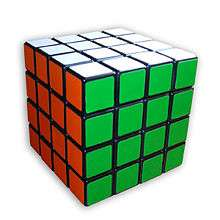Rubik's Revenge in solved state

The parity of a permutation (as defined in abstract algebra) is the parity of the number of transpositions into which the permutation can be decomposed. For example (ABC) to (BCA) is even because it can be done by swapping A and B then C and A (two transpositions). It can be shown that no permutation can be decomposed both in an even and in an odd number of transpositions. Hence the above is a suitable definition. In Rubik's Cube, Megaminx, and other twisting puzzles, the moves of the puzzle allow only even permutations of the puzzle pieces, so parity is important in understanding the configuration space of these puzzles.

The Feit–Thompson theorem states that a finite group is always solvable if its order is an odd number. This is an example of odd numbers playing a role in an advanced mathematical theorem where the method of application of the simple hypothesis of "odd order" is far from obvious.

### Analysis

The parity of a function describes how its values change when its arguments are exchanged with their negations. An even function, such as an even power of a variable, gives the same result for any argument as for its negation. An odd function, such as an odd power of a variable, gives for any argument the negation of its result when given the negation of that argument. It is possible for a function to be neither odd nor even, and for the case f(x) = 0, to be both odd and even. The Taylor series of an even function contains only terms whose exponent is an even number, and the Taylor series of an odd function contains only terms whose exponent is an odd number.

### Combinatorial game theory

In combinatorial game theory, an evil number is a number that has an even number of 1's in its binary representation, and an odious number is a number that has an odd number of 1's in its binary representation; these numbers play an important role in the strategy for the game Kayles. The parity function maps a number to the number of 1's in its binary representation, modulo 2, so its value is zero for evil numbers and one for odious numbers. The Thue–Morse sequence, an infinite sequence of 0's and 1's, has a 0 in position i when i is evil, and a 1 in that position when i is odious.

In information theory, a parity bit appended to a binary number provides the simplest form of error detecting code. If a single bit in the resulting value is changed, then it will no longer have the correct parity: changing a bit in the original number gives it a different parity than the recorded one, and changing the parity bit while not changing the number it was derived from again produces an incorrect result. In this way, all single-bit transmission errors may be reliably detected. Some more sophisticated error detecting codes are also based on the use of multiple parity bits for subsets of the bits of the original encoded value.

In wind instruments with a cylindrical bore and in effect closed at one end, such as the clarinet at the mouthpiece, the harmonics produced are odd multiples of the fundamental frequency. (With cylindrical pipes open at both ends, used for example in some organ stops such as the open diapason, the harmonics are even multiples of the same frequency for the given bore length, but this has the effect of the fundamental frequency being doubled and all multiples of this fundamental frequency being produced.) See harmonic series (music).

In some countries, house numberings are chosen so that the houses on one side of a street have even numbers and the houses on the other side have odd numbers. Similarly, among United States numbered highways, even numbers primarily indicate east-west highways while odd numbers primarily indicate north-south highways. Among airline flight numbers, even numbers typically identify eastbound or northbound flights, and odd numbers typically identify westbound or southbound flights.

1. A.V.Vijaya & Dora Rodriguez, Figuring Out Mathematics, Pearson Education India, pp. 20–21, ISBN 9788131703571.
2. Bóna, Miklós (2011), A Walk Through Combinatorics: An Introduction to Enumeration and Graph Theory, World Scientific, p. 178, ISBN 9789814335232.
3. Bassarear, Tom (2010), Mathematics for Elementary School Teachers, Cengage Learning, p. 198, ISBN 9780840054630.
4. Sidebotham, Thomas H. (2003), The A to Z of Mathematics: A Basic Guide, John Wiley & Sons, p. 181, ISBN 9780471461630.
5. Owen, Ruth L. (1992), "Divisibility in bases" (PDF), The Pentagon: A Mathematics Magazine for Students, 51 (2): 17–20.
6. Pólya, George; Tarjan, Robert E.; Woods, Donald R. (2009), Notes on Introductory Combinatorics, Springer, pp. 21–22, ISBN 9780817649524.
7. Tankha (2006), Ancient Greek Philosophy: Thales to Gorgias, Pearson Education India, p. 136, ISBN 9788177589399.
8. Froebel, Friedrich; Translator Josephine Jarvis (1885). The Education of Man. New York: A Lovell & Company. p. 240.
9. Conway, J. H.; Sloane, N. J. A. (1999), Sphere packings, lattices and groups, Grundlehren der Mathematischen Wissenschaften [Fundamental Principles of Mathematical Sciences], 290 (3rd ed.), New York: Springer-Verlag, p. 10, ISBN 0-387-98585-9, MR 1662447.
10. Pandolfini, Bruce (1995), Chess Thinking: The Visual Dictionary of Chess Moves, Rules, Strategies and Concepts, Simon and Schuster, pp. 273–274, ISBN 9780671795023.
11. Mendelsohn, N. S. (2004), "Tiling with dominoes", The College Mathematics Journal, 35 (2): 115–120, doi:10.2307/4146865, JSTOR 4146865.
12. Bruckner, Andrew M.; Bruckner, Judith B.; Thomson, Brian S. (1997), Real Analysis, p. 37, ISBN 0-13-458886-X.
13. Stillwell, John (2003), Elements of Number Theory, Springer, p. 199, ISBN 9780387955872.
14. Lial, Margaret L.; Salzman, Stanley A.; Hestwood, Diana (2005), Basic College Mathematics (7th ed.), Addison Wesley, p. 128, ISBN 9780321257802.
15. Dudley, Underwood (1992), "Perfect numbers", Mathematical Cranks, MAA Spectrum, Cambridge University Press, pp. 242–244, ISBN 9780883855072.
16. Oliveira e Silva, Tomás; Herzog, Siegfried; Pardi, Silvio (2013), "Empirical verification of the even Goldbach conjecture, and computation of prime gaps, up to 4·1018" (PDF), Mathematics of Computation, doi:10.1090/s0025-5718-2013-02787-1. In press.
17. Cameron, Peter J. (1999), Permutation Groups, London Mathematical Society Student Texts, 45, Cambridge University Press, pp. 26–27, ISBN 9780521653787.
18. Joyner, David (2008), "13.1.2 Parity conditions", Adventures in Group Theory: Rubik's Cube, Merlin's Machine, and Other Mathematical Toys, JHU Press, pp. 252–253, ISBN 9780801897269.
19. Bender, Helmut; Glauberman, George (1994), Local analysis for the odd order theorem, London Mathematical Society Lecture Note Series, 188, Cambridge: Cambridge University Press, ISBN 0-521-45716-5, MR 1311244; Peterfalvi, Thomas (2000), Character theory for the odd order theorem, London Mathematical Society Lecture Note Series, 272, Cambridge: Cambridge University Press, ISBN 0-521-64660-X, MR 1747393.
20. Gustafson, Roy David; Hughes, Jeffrey D. (2012), College Algebra (11th ed.), Cengage Learning, p. 315, ISBN 9781111990909.
21. Jain, R. K.; Iyengar, S. R. K. (2007), Advanced Engineering Mathematics, Alpha Science Int'l Ltd., p. 853, ISBN 9781842651858.
22. Guy, Richard K. (1996), "Impartial games", Games of no chance (Berkeley, CA, 1994), Math. Sci. Res. Inst. Publ., 29, Cambridge: Cambridge Univ. Press, pp. 61–78, MR 1427957. See in particular p. 68.
23. Bernhardt, Chris (2009), "Evil twins alternate with odious twins", Mathematics Magazine, 82 (1): 57–62, doi:10.4169/193009809x469084, JSTOR 27643161.
24. Moser, Stefan M.; Chen, Po-Ning (2012), A Student's Guide to Coding and Information Theory, Cambridge University Press, pp. 19–20, ISBN 9781107015838.
25. Berrou, Claude (2011), Codes and turbo codes, Springer, p. 4, ISBN 9782817800394.
26. Randall, Robert H. (2005), An Introduction to Acoustics, Dover, p. 181, ISBN 9780486442518.
27. Cromley, Ellen K.; McLafferty, Sara L. (2011), GIS and Public Health (2nd ed.), Guilford Press, p. 100, ISBN 9781462500628.
28. Swift, Earl (2011), The Big Roads: The Untold Story of the Engineers, Visionaries, and Trailblazers Who Created the American Superhighways, Houghton Mifflin Harcourt, p. 95, ISBN 9780547549132.
29. Lauer, Chris (2010), Southwest Airlines, Corporations that changed the world, ABC-CLIO, p. 90, ISBN 9780313378638.